Thermometer

The thermometer showed -3°C at morning. Then temperature was increased by 1°C again increased by 1°C and then decreased by 1°C and then decreased by 4°C.

Which terminal temperature thermometer shows?

Result

t =  -6 °C

Solution:Leave us a comment of example and its solution (i.e. if it is still somewhat unclear...):Be the first to comment!Next similar examples:

1. Thermometerthermometer showed -12 degrees Celsius at the morning then the temperature rises by 4 degrees and later again increased by 2 degrees at the evening has fallen by 5 degrees and then fall 3 degrees. What end temperature does the thermometer show?
2. This morningThis morning it was 80 degrees F outside the temperature increased 12 degrees F. What is the temperature now?
3. The temperarture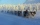The temperarture is 0 degrees. It drops 5 degrees after 1 hour and then drops 3 degrees more the next hour. What is the temperature after 6 hours?
4. Car parking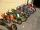The car park has 45 motorcycles and 25 cars. 14 cars went away and 2 arrived, then departs 12 motorcycles and 2 arrive. How many motorbikes and cars there are now?
5. Freezer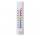Temperature is -8 degrees Celsius in the freezer. Outside temperature is 2 degrees Celsius. One minute took to change temperature by one degree Celsius if we open door. How many minutes the temperature in the freezer will rise?In about 12 hours in North Dakota the temperature rose from -33 degrees farenheit to 50 degrees farenheit. By how much did the temperature change?At a weather centre, the temperature at midnight was -2 degree Celsius and by noon it had raised 4 degree Celsius. What is the new temperature?
8. Change in temperatureStarting temperature is 21°C, the highest temperature is 32°C. What is the change in temperature?
9. Degrees 2The temperature was 3°F and falls four degrees Fahrenheit. What is actually temperature?
10. The temperatureThe temperature at 1:00 was 10 F. Between 1:00 and 2:00, the temperature dropped 15F. Between 2:00 and 3:00, the temperature rose 3F. What is the temperature at 3:00?
11. Numbers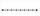Find number on thenumber line that has same distance from this numbers:
12. Roman numerals +Add up the number writtens in Roman numerals. Write the results as a decimal number.
13. Roman numerals 2+Add up the number writtens in Roman numerals. Write the results as a roman numbers.
14. Operations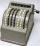Sum of the numbers 1.01 and 3.35 multiply by the difference of numbers 6.69 and 1.39.
15. Book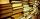Jitka read on holidays book that has 180 pages. In the first week read 45 pages. In the second week she read 15 pages more than the first week. How many pages left to read it yet?
16. Floods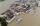After the floods that hit a school, parents help repair the damage. My father worked at the school 50 hours, my mom 5 hours less. How many hours worked mother? How many hours worked my parents together?
17. With bracket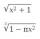Calculate (evaluate) simple mathematical expression with a negative numbers and a bracket: 13+15*5-2*(-6)